Students can download 11th Business Maths Chapter 1 Matrices and Determinants Ex 1.1 Questions and Answers, Notes, Samcheer Kalvi 11th Business Maths Guide Pdf helps you to revise the complete Tamilnadu State Board New Syllabus, helps students complete homework assignments and to score high marks in board exams.

## Tamilnadu Samacheer Kalvi 11th Business Maths Solutions Chapter 1 Matrices and Determinants Ex 1.1

### Samacheer Kalvi 11th Business Maths Matrices and Determinants Ex 1.1 Text Book Back Questions and Answers

Question 1.
Find the minors and cofactors of all the elements of the following determinants.
(i) $$\left|\begin{array}{cc} 5 & 20 \\ 0 & -1 \end{array}\right|$$
(ii) $$\left|\begin{array}{rrr} 1 & -3 & 2 \\ 4 & -1 & 2 \\ 3 & 5 & 2 \end{array}\right|$$
Solution:
(i) $$\left|\begin{array}{cc} 5 & 20 \\ 0 & -1 \end{array}\right|$$
Minor of 5 = M11 = -1
Minor of 20 = M12 = 0
Minor of 0 = M21 = 20
Minor of -1 = M22 = 5
Cofactor of 5 = A11 = (-1)1+1 M11 = 1 × -1 = -1
Cofactor of 20 = A12 = (-1)1+2 M12 = -1 × 0 = 0
Cofactor of 0 = A21 = (-1)2+1 M21 = -1 × 20 = -20
Cofactor of -1 = A22 = (-1)2+2 M22 = 1 × 5 = 5

(ii) $$\left|\begin{array}{rrr} 1 & -3 & 2 \\ 4 & -1 & 2 \\ 3 & 5 & 2 \end{array}\right|$$
Minor of 1 is M11 = $$\left|\begin{array}{rr} -1 & 2 \\ 5 & 2 \end{array}\right|$$ = -2 – 10 = -12
Minor of -3 is M12 = $$\left|\begin{array}{ll} 4 & 2 \\ 3 & 2 \end{array}\right|$$ = 8 – 6 = 2
Minor of 2 is M13 = $$\left|\begin{array}{rr} 4 & -1 \\ 3 & 5 \end{array}\right|$$ = 20 + 3 = 23
Minor of 4 is M21 = $$\left|\begin{array}{rr} -3 & 2 \\ 5 & 2 \end{array}\right|$$ = -6 – 10 = -16
Minor of -1 is M22 = $$\left|\begin{array}{ll} 1 & 2 \\ 3 & 2 \end{array}\right|$$ = 2 – 6 = -4
Minor of 2 is M23 = $$\left|\begin{array}{rr} 1 & -3 \\ 3 & 5 \end{array}\right|$$ = 5 + 9 = 14
Minor of 3 is M31 = $$\left|\begin{array}{cc} -3 & 2 \\ -1 & 2 \end{array}\right|$$ = -6 + 2 = -4
Minor of 5 is M32 = $$\left|\begin{array}{ll} 1 & 2 \\ 4 & 2 \end{array}\right|$$ = 2 – 8 = -6
Minor of 2 is M33 = $$\left|\begin{array}{cc} 1 & -3 \\ 4 & -1 \end{array}\right|$$ = -1 + 12 = 11
Cofactor of 1 is A11 = (-1)1+1 M11 = -12
Cofactor of -3 is A12 = (-1)1+2 M12 = -2
Cofactor of 2 is A13 = (-1)1+3 M13 = 23
Cofactor of 4 is A21 = (-1)2+1 M21 = -1 × -16 = 16
Cofactor of -1 is A22 = (-1)2+2 M22 = -4
Cofactor of 2 is A23 = (-1)2+3 M23 = -14
Cofactor of 3 is A31 = (-1)3+1 M31 = -4
Cofactor of 5 is A32 = (-1)3+2 M32 = -1 × -6 = 6
Cofactor of 2 is A33 = (-1)3+3 M33 = 11Question 2.
Evaluate $$\left|\begin{array}{rrr} 3 & -2 & 4 \\ 2 & 0 & 1 \\ 1 & 2 & 3 \end{array}\right|$$
Solution: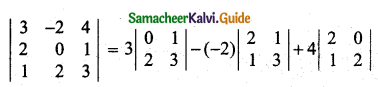= 3(0 – 2) + 2(6 – 1) + 4(4 – 0)
= -6 + 10 + 16
= 20

Question 3.
Solve: $$\left|\begin{array}{lll} 2 & x & 3 \\ 4 & 1 & 6 \\ 1 & 2 & 7 \end{array}\right|=0$$
Solution: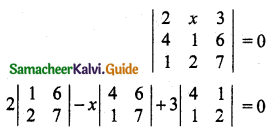2(7 – 12) – x(28 – 6) + 3(8 – 1) = 0
2(-5) – x(22) + 3(7) = 0
-10 – 22x + 21 = 0
-22x + 11 = 0
-22x = -11
x = $$\frac{-11}{-22}=\frac{1}{2}$$Question 4.
Find |AB| if A = $$\left[\begin{array}{rr} 3 & -1 \\ 2 & 1 \end{array}\right]$$ and B = $$\left[\begin{array}{rr} 3 & 0 \\ 1 & -2 \end{array}\right]$$
Solution: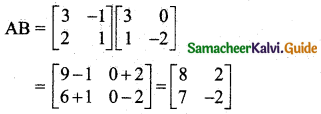∴ |AB| = $$\left|\begin{array}{rr} 8 & 2 \\ 7 & -2 \end{array}\right|$$ = -16 – 14 = -30

Question 5.
Solve: $$\left|\begin{array}{rrr} 7 & 4 & 11 \\ -3 & 5 & x \\ -x & 3 & 1 \end{array}\right|=0$$
Solution: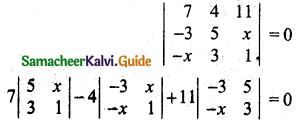7(5 – 3x) – 4(-3 + x2) + 11(-9 + 5x) = 0
35 – 21x + 12 – 4x2 – 99 + 55x = 0
-4x2 – 21x + 55x + 35 + 12 – 99 = 0
-4x2 + 34x – 52 = 0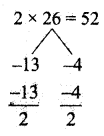Divide throughout by -2 we get
2x2 – 17x + 26 = 0
(2x – 13) (x – 2) = 0
2x – 13 = 0 (or) x – 2 = 0
x = $$\frac{13}{2}$$ (or) x = 2
∴ x = $$\frac{13}{2}$$, x = 2Question 6.
Evaluate: $$\left|\begin{array}{lll} 1 & a & a^{2}-b c \\ 1 & b & b^{2}-c a \\ 1 & c & c^{2}-a b \end{array}\right|$$
Solution:
$$\left|\begin{array}{lll} 1 & a & a^{2}-b c \\ 1 & b & b^{2}-c a \\ 1 & c & c^{2}-a b \end{array}\right|$$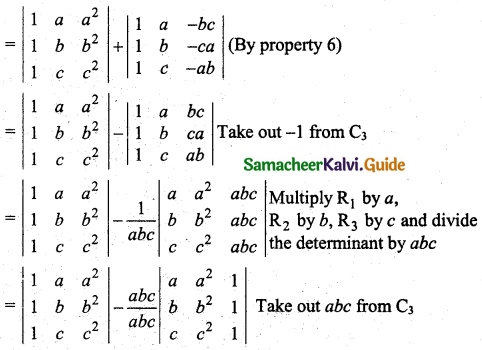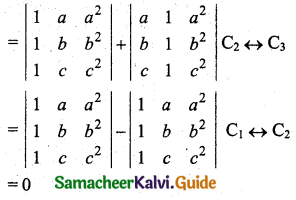Question 7.
Prove that $$\left|\begin{array}{lll} \frac{1}{a} & b c & b+c \\ \frac{1}{b} & c a & c+a \\ \frac{1}{c} & a b & a+b \end{array}\right|=0$$
Solution:
$$\left|\begin{array}{lll} \frac{1}{a} & b c & b+c \\ \frac{1}{b} & c a & c+a \\ \frac{1}{c} & a b & a+b \end{array}\right|$$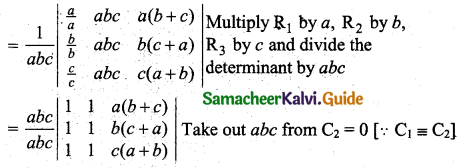Hence proved.Question 8.
Prove that $$\left|\begin{array}{ccc} -a^{2} & a b & a c \\ a b & -b^{2} & b c \\ a c & b c & -c^{2} \end{array}\right|=4 a^{2} b^{2} c^{2}$$
Solution:
$$\left|\begin{array}{ccc} -a^{2} & a b & a c \\ a b & -b^{2} & b c \\ a c & b c & -c^{2} \end{array}\right|$$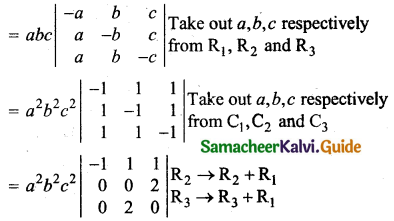= a2b2c2 [-(0 – 4) + 0 + 0]
= 4a2b2c2